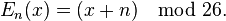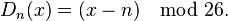# Caesar Cipher

In cryptography, a Caesar cipher, also known as shift cipher, Caesar's cipher, Caesar's code or Caesar shift, is one of the simplest and most widely known encryption techniques. It is a type of substitution cipher in which each letter in the plaintext is 'shifted' a certain number of places down the alphabet. For example, with a shift of 1, A would be replaced by B, B would become C, and so on. The method is named after Julius Caesar, who apparently used it to communicate with his generals.

More complex encryption schemes such as the vigenere cipher employ the Caesar cipher as one element of the encryption process. The widely known ROT13 'encryption' is simply a Caesar cipher with an offset of 13. As with all single-alphabet substitution ciphers, the Caesar cipher is easily broken and in modern practice offers essentially no communication security.

The encryption of Caesar cipher can be represented using modular arithmetic by first transforming the letters into numbers, according to the scheme, A = 0, B = 1,..., Z = 25. Encryption of a letter x by a shift n can be described mathematically as,Decryption is performed similarly,Example:

To pass an encrypted message from one person to another, it is first necessary that both parties have the 'key' for the cipher, so that the sender may encrypt it and the receiver may decrypt it. For the Caesar cipher, the key is the number of characters to shift the cipher alphabet.

Here is a quick example for the encryption and decryption of Caesar cipher. The text we will encrypt is 'cryptography', with a shift (key) of 3.

 Plain Text Alphabet Number + Key Cipher Text c r y p t o g r a p h y 2 + 3 17 + 3 24 + 3 15 + 3 19 + 3 14 + 3 6 + 3 17 + 3 0 + 3 15 + 3 7 + 3 24 + 3 f u b s w r j u d s k b

Decryption is just as easy, by using an offset of -3.

 Cipher Text Alphabet Number - Key Plain Text f u b s w r j u d s k b 5 - 3 20 - 3 1 - 3 18 - 3 22 - 3 17 - 3 9 - 3 20 - 3 3 - 3 18 - 3 10 - 3 1 - 3 c r y p t o g r a p h y

Security:

Caesar cipher is not a secure cryptosystem because there are only 26 possible keys to try out, we can simply try each possibility and see which one results in a piece of readable text. If you happen to know what a piece of the ciphertext is, or you can guess a piece, then this will allow you to immediately find the key.

If this is not possible, a more systematic approach is to match up the frequency distribution of the letters. By graphing the frequencies of letters in the ciphertext, and by knowing the expected distribution of those letters in the original language of the plaintext, a human can easily spot the value of the shift by looking at the displacement of particular features of the graph. This is known as frequency analysis. For example, in the English language the plaintext frequencies of the letters E, T, (usually most frequent), and Q, Z (typically least frequent) are particularly distinctive.

									private static char Cipher(char ch, int key)
{
if (!char.IsLetter(ch))
return ch;

char offset = char.IsUpper(ch) ? 'A' : 'a';
return (char)((((ch + key) - offset) % 26) + offset);
}

public static string Encipher(string input, int key)
{
string output = string.Empty;

foreach (char ch in input)
output += Cipher(ch, key);

return output;
}

public static string Decipher(string input, int key)
{
return Encipher(input, 26 - key);
}


### Example

									string text = "The quick brown fox jumps over the lazy dog";
string cipherText = Encipher(text, 3);
string plainText = Decipher(cipherText, 3);


### Output

									cipherText:	"Wkh txlfn eurzq ira mxpsv ryhu wkh odcb grj"
plainText:	"The quick brown fox jumps over the lazy dog"